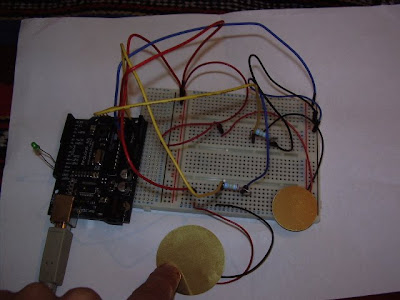## Tuesday 9 November 2010Two Piezos used as knock sensors.

Arduno code:
#include
const int buttonPin = 2; //DIGITAL 2const int ledPin = 13;int buttonState = 0; ///above = button stuff
int xCoord;int yCoord;char coords;
void setup(){ // initialize the LED pin as an output: pinMode(ledPin, OUTPUT); // initialize the pushbutton pin as an input: pinMode(buttonPin, INPUT); Serial.begin(9600);}

xCoord = analogRead(0); yCoord = analogRead(1); sprintf(coords, "%d,%d", xCoord, yCoord); Serial.println(coords); delay(50); }

Processing code
// based on Graph // by David A. Mellis
import processing.serial.*;
Serial port;String buff = "";String buff1 = "";String buff2 = "";//String buff3 = ""; //meint index = 0;int NEWLINE = 10;
// Store the last 256 values received so we can graph them.int[] valuesx = new int;int[] valuesy = new int;//int[] button_value = new int;//mevoid setup(){ size(512, 512);
port = new Serial(this, "COM7", 9600); // If you know the name of the port used by the Arduino board, you // can specify it directly like this. //port = new Serial(this, "COM1", 9600);
}
void draw(){ background(250);
stroke(0); // Graph the stored values by drawing a lines between them. for (int i = 0; i <> 0){ serialEvent(port.read()); } // image(etch,0, 0, width, height);}
void serialEvent(int serial){ if (serial != NEWLINE) { // Store all the characters on the line. buff += char(serial); } else { // The end of each line is marked by two characters, a carriage // return and a newline. We're here because we've gotten a newline, // but we still need to strip off the carriage return. buff = buff.substring(0, buff.length()-1); index = buff.indexOf(","); buff1 = buff.substring(0, index); buff2 = buff.substring(index+1, buff.length()); // Parse the String into an integer. We divide by 4 because // analog inputs go from 0 to 1023 while colors in Processing // only go from 0 to 255. int x = Integer.parseInt(buff1)/2; int y = Integer.parseInt(buff2)/2;
// Clear the value of "buff" buff = ""; // Shift over the existing values to make room for the new one. for (int i = 0; i < font =" loadFont(" mychannel="new" mychannel="new" mychannel="new" mychannel="new" mychannel="new" mychannel="new" mychannel="new" mychannel2="new" mychannel3="new" rando =" (int)random(1," rando ="=" rando ="=" rando ="=" mousepressed ="=">